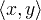# Linear Algebra Symbols

a ∙ b
a × b
A ⊗ B

Symbol
Symbol Name
Meaning / definition
dot
scalar product
×
cross
vector product
AB
tensor product
tensor product of A and Binner product
[ ]
brackets
matrix of numbers
( )
parentheses
matrix of numbers
| A |
determinant
determinant of matrix A
det(A)
determinant
determinant of matrix A
|| x ||
double vertical bars
norm
A
Hermitian matrix
matrix conjugate transpose
A -1
inverse matrix
A A-1 = I
rank(A)
matrix rank
rank of matrix A
dim(U)
dimension
dimension of matrix A